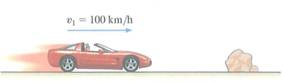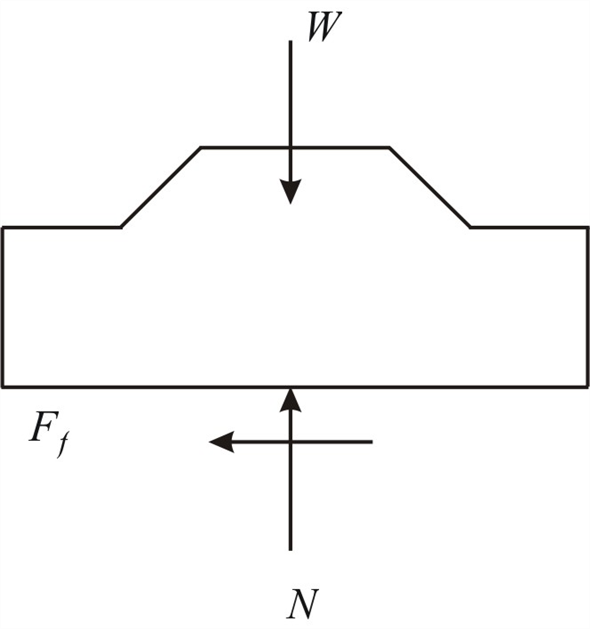Problem

# The 2-Mg car has a velocity of v1 = 100 km/h when the driver sees an obstacle in front of...

The 2-Mg car has a velocity of v1 = 100 km/h when the driver sees an obstacle in front of the car. It takes 0.75 s for him to react and lock the brakes, causing the car to skid. If the car stops when it has traveled a distance of 175 m, determine the coefficient of kinetic friction between the tires and the road.#### Step-by-Step Solution

Solution 1

The driver of a car sees an obstacle and applies brakes, causing the car to skid Mass of the car, $$m=2000 \mathrm{~kg}$$ Initial velocity of the car, $$v_{1}=100 \mathrm{~km} / \mathrm{h}$$ $$=27.778 \mathrm{~m} / \mathrm{s}$$

Final velocity of the car, $$v_{2}=0 \mathrm{~m} / \mathrm{s}$$

Time taken to react and apply brakes, $$t=0.75 \mathrm{~s}$$

Before car stops, distance traveled by car $$d=175 \mathrm{~m}$$

Coefficient of kinetic friction between the tires and the road is $$\mu_{2}$$

Free-body diagram of car:Let $$F_{f}$$ is the frictional force, which is acting opposite to the motion of the car

$$F_{f}=\mu_{k} N$$

Equations of motion:

\begin{aligned} &+\uparrow \sum F_{y}=m a_{y} \\ &N-W=m a_{y} \\ &N-m g=m(0) \\ &N=m g \\ &N=2000 \times 9.81 \\ &N=19620 \mathrm{~N} \end{aligned}

Substituting value of $$\mathrm{N}$$ in $$F_{f}=\mu_{k} N$$

\begin{aligned} &F_{f}=\mu_{h} \times 19620 \\ &F_{f}=19620 \mu_{k} \end{aligned}

A force does work when it moves through a displacement in the direction of the force. Here frictional force is acting in opposite direction to the displacement of work. So $$F_{f}$$ does negative work. Normal force $$N$$, weight w does no work since they never undergoes displacement along its line of action.

Principle of work and energy:

Applying the work-energy principle

$$T_{1}+\sum U_{1-2}=T_{2} \quad--(1)$$

Where, $$T_{1}$$ - initial kinetic energy

$$T_{2}-$$ Final kinetic energy

$$\sum U_{1-2}-$$ Work done by all forces

Substitute the known values in equation (1)

$$\frac{1}{2} m v_{1}^{2}-\left(F_{f} \times d^{\prime}\right)=\frac{1}{2} m v_{2}^{2}$$

Where, $$d^{\prime}$$ is the skidding distance of the car

\begin{aligned} &\left(\frac{1}{2} \times 2000 \times 27.778^{2}\right)-\left(19620 \mu_{k} \times d^{\prime}\right)=\frac{1}{2} m \times 0^{2} \\ &19620 \mu_{k} d^{\prime}=771617.284 \\ &d^{\prime}=\frac{39.328}{\mu_{k}} \end{aligned}

Car's brakes are applied after $$0.75 \mathrm{~s}$$

The distance traveled by the car during the reaction time, $$d^{\prime \prime}=v_{1} t$$

\begin{aligned} &d^{\prime \prime}=27.778 \times 0.75 \\ &d^{\prime \prime}=20.83 \mathrm{~m} \end{aligned}

Thus the total distance traveled by car before it stops is $$d=d^{\prime}+d^{*}$$

\begin{aligned} &175=\frac{39.327}{\mu_{k}}+20.83 \\ &\frac{39.327}{\mu_{k}}=154.17 \\ &\mu_{k}=\frac{39.327}{154.17} \\ &\mu_{k}=0.255 \end{aligned}